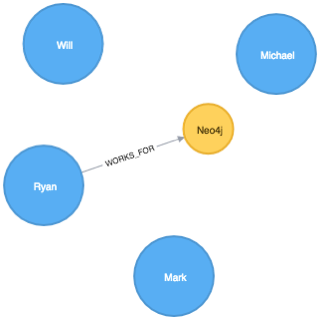# Neo4j: How do null values even work?

Every now and then I find myself wanting to import a CSV file into Neo4j and I always get confused with how to handle the various null values that can lurk within.

Let's start with an example that doesn't have a CSV file in sight. Consider the following list and my attempt to only return null values:

``````
WITH [null, "null", "", "Mark"] AS values
UNWIND values AS value
WITH value WHERE value = null
RETURN value

(no changes, no records)
``````

Hmm that's weird. I'd have expected that at least keep the first value in the collection. What about if we do the inverse?

``````
WITH [null, "null", "", "Mark"] AS values
UNWIND values AS value
WITH value WHERE value <> null
RETURN value

(no changes, no records)
``````

Still nothing! Let's try returning the output of our comparisons rather than filtering rows:

``````
WITH [null, "null", "", "Mark"] AS values
UNWIND values AS value
RETURN value = null AS outcome

╒═══════╤═════════╕
│"value"│"outcome"│
╞═══════╪═════════╡
│null   │null     │
├───────┼─────────┤
│"null" │null     │
├───────┼─────────┤
│""     │null     │
├───────┼─────────┤
│"Mark" │null     │
└───────┴─────────┘
``````

Ok so that isn't what we expected. Everything has an 'outcome' of 'null'! What about if we want to check whether the value is the string "Mark"?

``````
WITH [null, "null", "", "Mark"] AS values
UNWIND values AS value
RETURN value = "Mark" AS outcome

╒═══════╤═════════╕
│"value"│"outcome"│
╞═══════╪═════════╡
│null   │null     │
├───────┼─────────┤
│"null" │false    │
├───────┼─────────┤
│""     │false    │
├───────┼─────────┤
│"Mark" │true     │
└───────┴─────────┘
``````

From executing this query we learn that if one side of a comparison is null then the return value is always going to be null.

So how do we exclude a row if it's null?

It turns out we have to use the 'is' keyword rather than using the equality operator. Let's see what that looks like:

``````
WITH [null, "null", "", "Mark"] AS values
UNWIND values AS value
WITH value WHERE value is null
RETURN value

╒═══════╕
│"value"│
╞═══════╡
│null   │
└───────┘
``````

And the positive case:

``````
WITH [null, "null", "", "Mark"] AS values
UNWIND values AS value
WITH value WHERE value is not null
RETURN value

╒═══════╕
│"value"│
╞═══════╡
│"null" │
├───────┤
│""     │
├───────┤
│"Mark" │
└───────┘
``````

What if we want to get rid of empty strings?

``````
WITH [null, "null", "", "Mark"] AS values
UNWIND values AS value
WITH value WHERE value <> ""
RETURN value

╒═══════╕
│"value"│
╞═══════╡
│"null" │
├───────┤
│"Mark" │
└───────┘
``````

Interestingly that also gets rid of the null value which I hadn't expected. But if we look for values matching the empty string:

``````
WITH [null, "null", "", "Mark"] AS values
UNWIND values AS value
WITH value WHERE value = ""
RETURN value

╒═══════╕
│"value"│
╞═══════╡
│""     │
└───────┘
``````

It's not there either! Hmm what's going on here:

``````
WITH [null, "null", "", "Mark"] AS values
UNWIND values AS value
RETURN value, value = "" AS isEmpty, value <> "" AS isNotEmpty

╒═══════╤═════════╤════════════╕
│"value"│"isEmpty"│"isNotEmpty"│
╞═══════╪═════════╪════════════╡
│null   │null     │null        │
├───────┼─────────┼────────────┤
│"null" │false    │true        │
├───────┼─────────┼────────────┤
│""     │true     │false       │
├───────┼─────────┼────────────┤
│"Mark" │false    │true        │
└───────┴─────────┴────────────┘
``````

null values seem to get filtered out for every type of equality match unless we explicitly check that a value 'is null'.

So how do we use this knowledge when we're parsing CSV files using Neo4j's LOAD CSV tool?

Let's say we have a CSV file that looks like this:

``````
\$ cat nulls.csv
name,company
"Mark",
"Michael",""
"Will",null
"Ryan","Neo4j"
``````

So none of the first three rows have a value for 'company'. I don't have any value at all, Michael has an empty string, and Will has a null value. Let's see how LOAD CSV interprets this:

``````
RETURN row

╒═════════════════════════════════╕
│"row"                            │
╞═════════════════════════════════╡
│{"name":"Mark","company":null}   │
├─────────────────────────────────┤
│{"name":"Michael","company":""}  │
├─────────────────────────────────┤
│{"name":"Will","company":"null"} │
├─────────────────────────────────┤
│{"name":"Ryan","company":"Neo4j"}│
└─────────────────────────────────┘
``````

We've got the full sweep of all the combinations from above. We'd like to create a Person node for each row but only create a Company node and associated 'WORKS_FOR' relationshp if an actual company is defined - we don't want to create a null company.

So we only want to create a company node and 'WORKS_FOR' relationship for the Ryan row.

The following query does the trick:

``````
MERGE (p:Person {name: row.name})
WITH p, row
WHERE row.company <> "" AND row.company <> "null"
MERGE (c:Company {name: row.company})
MERGE (p)-[:WORKS_FOR]->(c)

Added 5 labels, created 5 nodes, set 5 properties, created 1 relationship, statement completed in 117 ms.
``````

And if we visualise what's been created:Perfect. Perhaps this behaviour is obvious but it always trips me up so hopefully it'll be useful to someone else as well!

There's also a section on the Neo4j developer pages describing even more null scenarios that's worth checking out.